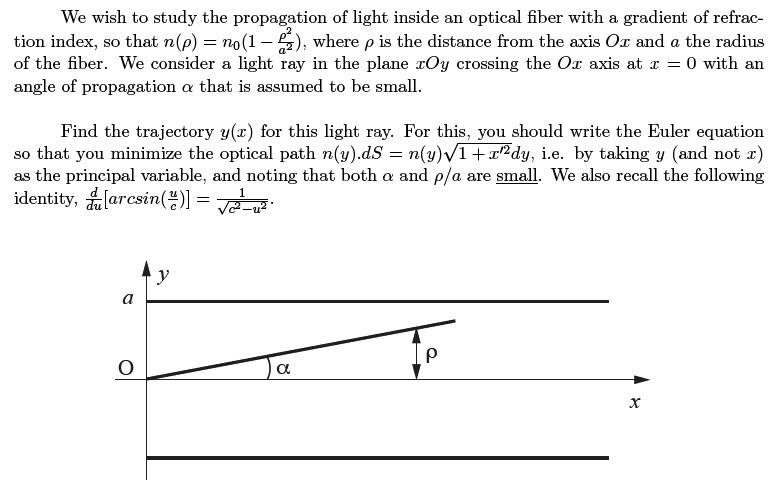# Light in an optical fiber - variational calculus

physman55

## Homework Statement## Homework Equations

$$\frac{\delta{F}}{\delta{y}} - \frac{d}{dx}\frac{\delta{F}}{\delta{y'}} = 0$$

## The Attempt at a Solution

I'm having trouble setting this one up. If I let the functional be

$$F(x,x',y) = n(y)\sqrt{1+(x')^2}$$

Applying the LE equation I obtain:

$$\frac{d}{dy}\frac{x'}{\sqrt{1+(x')^2}}n_0(1-\frac{y^2}{a^2})=0$$

And this is where I stopped (I haven't used either of the hints). Any hints?

physman55
Any ideas please? If p/a is small then I could write n(y)=n_0; but then I'm minimizing path length... so the answer is a straight line. Obviously this problem couldn't be that easy.

sgd37
it tells you y should be the principal variable so your functional should read

$$F(y,y')$$= $$n(y)$$$$\sqrt{1+y'^{2}}$$ and then calculate the EL equations

by my calculations you should get

$$\frac{\partial}{\partial x}$$$$(\frac{y'}{\sqrt{1+y'^{2}}})$$= $$-2\frac{y}{a^{2}}\sqrt{1+y'^{2}}$$

disregarding the (y/a)^2 term

Last edited:
physman55
By "y" being the principal value my prof means that "y" is the independent variable.

For the last line, why did you ignore n(y) when you took the partial with respect to y' (on the left)?

sgd37
yeah Y is the degree of freedom and the Lagrangian are always expressed in term of the degrees of freedom L(q,q') and this makes more sense since you are asked to find y(x)

I didn't ignore the n(y) its just that y is comparible to $$\rho$$ and since $$\frac{\rho}{a}$$ is small n(y) = n0

physman55
Ok thanks for the explanation, that makes sense. But shouldn't the d/dx be a total derivative and not a partial; and secondly; how the hell do you solve that ODE?

sgd37
i'm not sure about the entire thing but since dy is comparable to rho you can deduce that y' is approximate to alpha and thus all the y'^2 terms are negligible and you get the resulting ODE

$$y''=\frac{-2y}{a^{2}}$$

which has solution

$$Acos(\frac{\sqrt{2}x}{a}) +Bsin(\frac{\sqrt{2}x}{a})$$ which kind of makes sense since you expect it to bounce off the walls in a periodic fashion